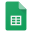# Discussion Board

There was a Recent Google Plus Discussion in which the teacher wanted to create a 'Discussion Board' in Google Classroom. The eventual answer was that it is possible to use the Question Feature of Classroom and allow students to respond to each other and have a stream that the teacher could use to grade the student discussion.

But in the meantime I decided to see if it was possible to create something within a google form that would allow the students to see and take part of the discussion and give the teacher a document of said discussion. So using Doc Appender, I have been able to create a project that might be useful for some to use.

To use this successfully as a discussion board, you will need to open two windows side by side. In the first window open the form, and in the second window open the Discussion Responses Spreadsheet, and all three documents so you can track in real time what is happening as you submit the form.

Here is the query for the 'All Discussions' tab.

``=SORT(QUERY(Discussion, "select A,B,C,D,E"),1,False)``

After I made the video, I added a sheet for each discussion so the teacher and students can look at all the comments, or just the comments for a question, or comments by teacher or student.

Here is the query for a sheet to just show the Current Events responses. I Duplicate this sheet, rename the sheet and change the formula to a different discussion.

``=SORT(QUERY(Discussion, "select A,B,C,D,E where B = 'Current Events'"),1,False) ``

I also duplicated these sheets and changed the formula so I have a sheet for each student.

``=SORT(QUERY(Discussion, "select A,B,C,D,E where C = 'Teacher'"),1,False) ``

I have added a grading component as well. In this case, each response by a student is either worth 1 or 0. Therefore the formula for the grade is where the G\$1 is the student name in the header (makes the formula more portable).

• `=SUMIF(\$C\$2:\$C,G\$1,\$F\$2:\$F)/COUNTIF(\$C\$2:\$C,G\$1)`

# THE SHEETDiscussion (Responses)